Related Articles
Class 8 NCERT Solutions – Chapter 11 Mensuration – Exercise 11.3
• Last Updated : 26 Nov, 2020

### Question 1. There are two cuboidal boxes as shown in the adjoining figure. Which box requires the lesser amount of material to make?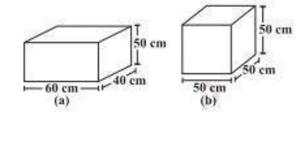Solution:

(a) For finding which box requires the lesser amount of material to make we have to calculate total surface area of both these boxes.

So, Length of first cuboidal box (l) = 60 cm

Breadth of first cuboidal box (b) = 40 cm

Height of first cuboidal box (h) = 50 cm

Total surface area of first cuboidal box = 2 × (lb + bh + hl)

= 2 × (60 × 40 + 40 × 50 + 50 × 60)

= 2 × (2400 + 2000 + 3000)

= 14800 cm2

Total surface area of first cuboidal box is 14800 cm².

(b) Also,

Length of second cubical box (l) = 50 cm

Breadth of second cubicalbox (b) = 50 cm

Height of second cubicalbox (h) = 50 cm

Total surface area of second cubical box = 6 (side)2

= 6 (50 × 50)

= 6 × 2500

= 15000 cm²

Total surface area of the second cubical box is 15000 cm2

From both of these result we found that cuboidal box (observation “a”) requires the lesser amount of material to make.

### Question 2. A suitcase measures 80 cm × 48 cm × 24 cm is to be covered with a tarpaulin cloth. How many meters of tarpaulin of width 96 cm is required to cover 100 such suitcases?

Solution:

Length of suitcase box (l)= 80 cm,

Breadth of suitcase box (b) = 48 cm

Height of cuboidal box (h) = 24 cm

Total surface area of suitcase box = 2 × (lb + bh + hl)

= 2 × (80 × 48 + 48 × 24 + 24 × 80)

= 2 × (3840 + 1152 + 1920)

= 2 × 6912

= 13824 cm²

Hence, Total surface area of suitcase box is 13824 cm²

As suitcase is fully covered by tarpaulin

Area of Tarpaulin cloth = Surface area of suitcase

(l × b) = 13824

(l × 96) = 13824

l = 144m

Required tarpaulin for 100 suitcases = 144 × 100 = 14400 cm = 144 m

Hence, tarpaulin cloth required to cover 100 suitcases is 144 m.

### Question 3. Find the side of a cube whose surface area is 600 cm².

Solution:

Given that

Surface area of cube = 600 cm²

Formula for surface area of a cube = 6(side)²

Substituting the values, we get

6 × (side)² = 600

(side)² = 100

Or side = ±10

We can’t take side as negative

Hence, the measure of each side of a cube is 10 cm

### Question 4. Rukhsar painted the outside of the cabinet of measure 1 m × 2 m × 1.5 m. How much surface area did she cover if she painted all except the bottom of the cabinet.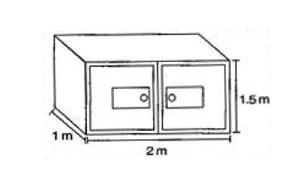Solution:

Length of cabinet (l) = 2 m,

Breadth of cabinet (b) = 1 m,

and Height of cabinet (h) = 1.5 m

Surface area of cabinet = 2 × (lb +  bh + hl) – lb

= 2 × (2 × 1 + 1 × 1.5 + 1.5 × 2)  – 2 × 1

= 2 × (2 +  1.5 + 3.0) – 2

= 2 × (6.5) – 2

= 13 – 2

= 11m²

Required surface area of cabinet is 11m²

### Question 5. Daniel is painting the walls and ceiling of a cuboidal hall with length, breadth and height of 15 m, 10 m and 7 m respectively. From each can of paint 100 m² of area is painted.How many cans of paint will she need to paint the room?

Solution:

Length of wall (l) = 15 m,

Breadth of wall (b) = 10 m

Height of wall, (h) = 7 m

Total Surface area of classroom = 2 × (lb + bh + hl) – lb

= 2 × (15 × 10 +  10 × 7 + 7 × 15) – (15 × 10)

= 2 × (150 + 70 + 105) – 150

= 650 – 150

= 500

Now, Required number of cans = Area of hall/Area of one can

= 500/100 = 5

Therefore, 5 cans are required to paint the room.

### Question 6. Describe how the two figures at the right are alike and how they are different. Which box has a larger lateral surface area?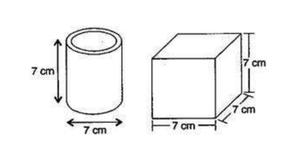Solution:

Given that,

Diameter of cylinder = 7 cm

Radius of cylinder (r) = 7 / 2 cm

Height of cylinder (h) = 7 cm

Surface area of cylinder = 2πrh

= 2 × (22 / 7) × (7 / 2) × 7 = 154

So, Lateral surface area of cylinder is 154 cm²

Now, lateral surface area of cube = 4 (side)² = 4 × 72 = 4 × 49 = 196

Lateral surface area of cube is 196 cm²

Hence, the cube has larger lateral surface area.

### Question 7. A closed cylindrical tank of radius 7 m and height 3 m is made from a sheet of metal. How many sheets of metal is required?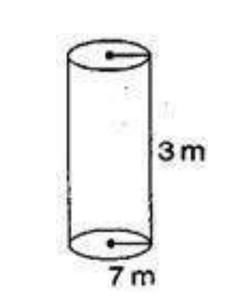Radius of cylindrical tank (r) = 7 m

Height of cylindrical tank (h) = 3 m

Total surface area of cylindrical tank = 2πrh + 2πr² = 2πr × (h + r)

= 2 × (22 / 7) × 7 (3 + 7)

= 44 × 10 = 440

Therefore, 440 m² metal sheet is required.

### Question 8.  The lateral surface area of a hollow cylinder is 4224 cm² It is cut along its height and formed a rectangular sheet of width 33 cm. Find the perimeter of the rectangular sheet?

Solution:

Lateral surface area of hollow cylinder = 4224 cm²

Height of hollow cylinder, h = 33 cm

and say r be the radius of the hollow cylinder

Curved surface area of hollow cylinder = 2πrh

4224 = 2 × π × r × 33

r = (4224) / (2π × 33)

r = 64 × 7/ 22

Now, Length of rectangular sheet, l = 2πr

l = 2 × 22 / 7× (64 × 7/ 22) = 128

So the length of the rectangular sheet is 128 cm.

Also, Perimeter of rectangular sheet = 2 × (l + b)

= 2 × (128 + 33)

= 322

The perimeter of rectangular sheet is 322 cm.

### Question 9. A road roller takes 750 complete revolutions to move once over to level a road. Find the area of the road if the diameter of a road roller is 84 cm and length is 1 m.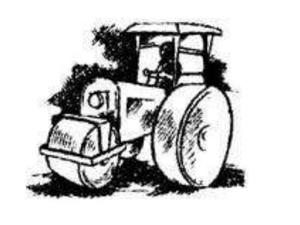Solution:

Diameter of road roller (d) = 84 cm

Radius of road roller (r) = d / 2 = 84 / 2 = 42 cm

Length of road roller (h) = 1 m = 100 cm

Curved surface area of road roller = 2πrh

= 2 × (22 / 7) × 42 × 100 = 26400

Curved surface area of road roller is 26400 cm²

Again, Area covered by road roller in 750 revolutions = 26400 × 750cm²

= 1,98,00,000 cm²

= 1,98,00,000 × (1 / 10,000) m² (1cm²= 1 / 10,000m²)

Hence, the area of the road is 1980 m².

### Question 10. A company packages its milk powder in cylindrical container whose base has a diameter of 14 cm and height 20 cm. Company places a label around the surface of the container (as shown in the figure). If the label is placed 2 cm from top and bottom, what is the area of the label?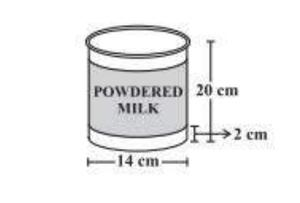Solution:

Diameter of cylindrical container (d) = 14 cm

Radius of cylindrical container (r) = d / 2 = 14 / 2 = 7 cm

Height of cylindrical container (H) = 20 cm

Height of the label (h) = Height of container – free space

= 20 – 2 (2)

= 16 cm

Area of label = 2πrh

= 2 × (22 / 7) × 7 × 16

= 704 cm²

Hence, the area of the label is 704 cm².

My Personal Notes arrow_drop_up